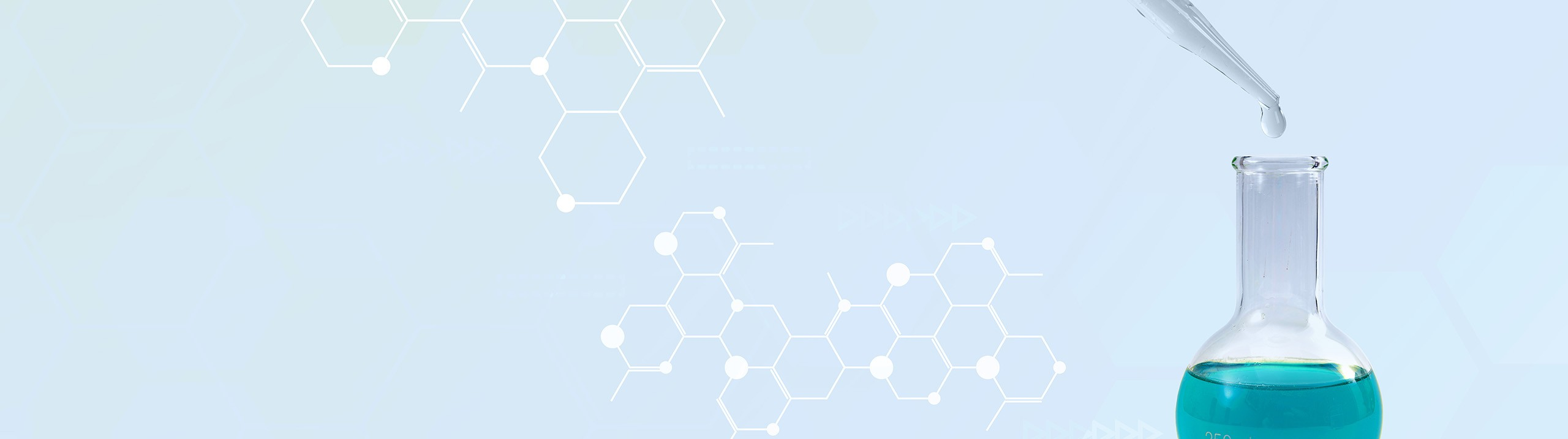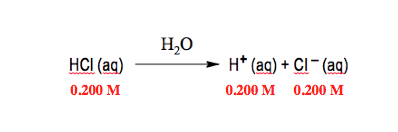## Analytical Chemistry

Learn the toughest concepts covered in your Analytical Chemistry class with step-by-step video tutorials and practice problems.

# General Approach to Acid-Base Systems

The Systematic Approach
1
concept

## Systematic Approach2m
Play a video:
recall that strong acids and strong bases are considered to be strong electrolytes. That means that when we place them in solution they will completely ionized within the solvent. Now we're gonna say in general remember we also said that the larger the K. A. Value for an acid than the stronger the acid will be and the greater the concentration of H plus ions, we could also say the larger the KB value, the stronger the base and therefore the larger the O. H minus concentration. Now here for example, we have hydrochloric acid as an example here when it's thrown into water are solvent, it completely ionizing into H plus ion and cl minus ion because it is a strong acid, there's 100% ionization. So the initial concentration of my acid represents the final concentrations of the ions form because again it's 100% ionization. Now strong acids and strong bases are both strong species. Of course if we're taking a look at acids, the fact that these acids are all strong, they're gonna have K. Values that are greater than one. So we can easily see that when we look at each of them, all of them give me a value greater than one or equal to one in the case of caloric acid. Now we would normally say that if we take a look at the concentration of my strong acid or strong base we can just take the negative log of that concentration to find P. H. Or P. O. H. Respectively. Now that's not entirely true. And even with the strong species we have to be mindful of the initial concentration given to us based on the initial concentration will have to take different approaches and understand different methods to find the correct ph and P. O. H. So take a look at the next video and see how we break down the different concentrations of strong acids and strong bases to help us determine the best course of action in order to find our P. H. Or R P O. H, respectively.2
concept

## Systematic Approach4m
Play a video:
we've been under the assumption that whenever we're given the concentration of a strong acid or strong base, we could take the negative log of those concentrations to find P. H. Or P. O. H. Respectively. Now, in actuality when calculating the ph of a solution, we must take into consideration the concentration of the strong acid or the strong base because depending on the concentrations given, we'll have to use different methods to find the correct ph and P. O. H. Respectively. Now here we split it up into three categories here for the first one when the concentration of your strong acid or strong base is equal to or greater than 10 to the negative six molar, that means that there's a significant amount of those two species that we can just simply look at their concentrations and take the negative log. So for example here we have nitric acid, it completely lionizes into H plus ion and nitrate ion because the concentration of 1.5 times 10 to negative three moller is greater than 10 to the negative six. We can just simply take the negative log of this concentration because remember, nitric acid being a strong acid completely breaks up into H plus ion. So this would be 100% generation of this ion. I would take the negative log of that concentration And that would give me my ph which come out which comes out to 2.82. Here we have sodium hydroxide again, the concentration as long as it's equal to or greater than 10 to the negative six Mohler, we have complete ionization and here we have 100% generation of this O. H minus ion here we can just simply take the negative log of this concentration. But now it gives me P O. H. So I plug that value in That give me 3.12. And then remember at this point PH equals 14 minus that value. So we'd get 10.88 at this point. So as long as my concentration is equal to or greater than 10 to the negative six, the concentration of the strong acid and strong base are significant enough to change the ph of my solution. So I can simply look at their concentrations and take the negative log to find P. H. Or P. O. H respectively. Now when the concentration that they have is equal to or less than 10 to the negative eight moller. And really we're going to say when it's less than 10 to the negative eight moller, then we're gonna say that the concentrations are not significant enough. Therefore the ph will be seven. That's because there isn't enough strong acid or enough strong base in order to change the ph of the solution as a whole. So here we have 10 to the negative 11 and 10 to the negative nine, they're not significant enough. So both solutions will be neutral. So again it's when concentrations are less than 10 to the negative eight moller. Finally, when you have your concentration between 10 to the negative, 6 to 10 to the negative eight moller. We're gonna take a systematic approach to finding our ph that's because at these concentrations they're not large enough but not small enough. So they will have some changing of the ph of our solution here. We have to be mindful that between these concentration values we have to take into account the auto ionization of water. Remember the auto ionization of water would produce some H Plus ion and some O. H minus ion. These concentrations would actually contribute to the overall concentrations of H plus and O H minus. Taking them into consideration is key to getting the correct ph for our strong acid and the correct P. O. H. For our strong base. So remember when it comes to strong acids and strong bases, they are strong electrolytes that completely ionized in water. But we have to be careful when looking at their concentrations depending where their concentration lies. Means we'll have to take one of three possible routes to find P. H. Or P. O. H. Respectively. So just keep in mind some of the tips that we went over here. And as we continue with this topic, keep in mind the strategies needed to find again your P. H. Or P. O. H.

Whenever the concentration of your strong acid or strong base is between 10-6 to 10-8 M a systematic approach may have to be used to determine pH or pOH.

3
example

## Systematic Approach Calculations8m
Play a video: